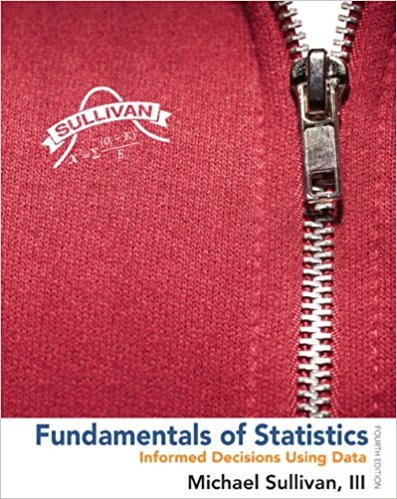×
×

# Solutions for Chapter Chapter C.4: Fundamentals of Statistics 4th Edition## Full solutions for Fundamentals of Statistics | 4th Edition

ISBN: 9780321838704Solutions for Chapter Chapter C.4

Solutions for Chapter Chapter C.4
4 5 0 405 Reviews
26
3
##### ISBN: 9780321838704

Fundamentals of Statistics was written by and is associated to the ISBN: 9780321838704. This textbook survival guide was created for the textbook: Fundamentals of Statistics, edition: 4. Since 31 problems in chapter Chapter C.4 have been answered, more than 290055 students have viewed full step-by-step solutions from this chapter. This expansive textbook survival guide covers the following chapters and their solutions. Chapter Chapter C.4 includes 31 full step-by-step solutions.

Key Statistics Terms and definitions covered in this textbook
• `-error (or `-risk)

In hypothesis testing, an error incurred by rejecting a null hypothesis when it is actually true (also called a type I error).

• a-error (or a-risk)

In hypothesis testing, an error incurred by failing to reject a null hypothesis when it is actually false (also called a type II error).

• Analysis of variance (ANOVA)

A method of decomposing the total variability in a set of observations, as measured by the sum of the squares of these observations from their average, into component sums of squares that are associated with speciic deined sources of variation

• Assignable cause

The portion of the variability in a set of observations that can be traced to speciic causes, such as operators, materials, or equipment. Also called a special cause.

• Average

See Arithmetic mean.

• Axioms of probability

A set of rules that probabilities deined on a sample space must follow. See Probability

• Backward elimination

A method of variable selection in regression that begins with all of the candidate regressor variables in the model and eliminates the insigniicant regressors one at a time until only signiicant regressors remain

• Bimodal distribution.

A distribution with two modes

• Binomial random variable

A discrete random variable that equals the number of successes in a ixed number of Bernoulli trials.

• Conditional probability distribution

The distribution of a random variable given that the random experiment produces an outcome in an event. The given event might specify values for one or more other random variables

• Conidence coeficient

The probability 1?a associated with a conidence interval expressing the probability that the stated interval will contain the true parameter value.

• Contrast

A linear function of treatment means with coeficients that total zero. A contrast is a summary of treatment means that is of interest in an experiment.

• Correlation

In the most general usage, a measure of the interdependence among data. The concept may include more than two variables. The term is most commonly used in a narrow sense to express the relationship between quantitative variables or ranks.

• Cumulative normal distribution function

The cumulative distribution of the standard normal distribution, often denoted as ?( ) x and tabulated in Appendix Table II.

• Degrees of freedom.

The number of independent comparisons that can be made among the elements of a sample. The term is analogous to the number of degrees of freedom for an object in a dynamic system, which is the number of independent coordinates required to determine the motion of the object.

• Discrete uniform random variable

A discrete random variable with a inite range and constant probability mass function.

• Eficiency

A concept in parameter estimation that uses the variances of different estimators; essentially, an estimator is more eficient than another estimator if it has smaller variance. When estimators are biased, the concept requires modiication.

• Estimator (or point estimator)

A procedure for producing an estimate of a parameter of interest. An estimator is usually a function of only sample data values, and when these data values are available, it results in an estimate of the parameter of interest.

• Exponential random variable

A series of tests in which changes are made to the system under study

• Fractional factorial experiment

A type of factorial experiment in which not all possible treatment combinations are run. This is usually done to reduce the size of an experiment with several factors.

×